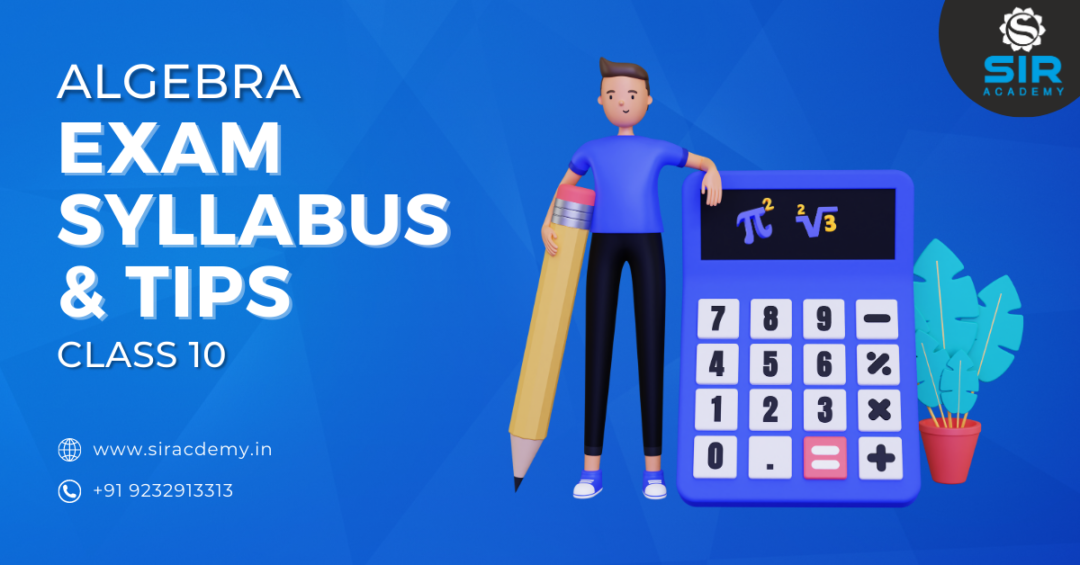# Algebra Study Material

Are you looking for an overall guide to know everything about your subject of class 10 and score good grades at the same time? You are at the right place here! Get access to the most Required Study Materials and Exam Preparation guide drafted for you exclusively by “Sir Academy” Download the Previous year Question papers and get a better understanding of the topics. In this blog we will discuss the Study Materials and Algebra exam syllabus for SSC board 2023-2024.

### Books to refer:

The resources you have and the way you use them determines how well you will Score in your exams.

If you are a CBSE Student, NCERT Textbooks should definitely be on your list. Apart from this, you can refer various textbooks namely,

• Mathematics for Class 10 by R D Sharma
• Secondary School Mathematics for Class 10 by RS Aggarwal
• All in one Mathematics by Arihant

For the SSC board,you can refer to your Textbooks, Digest as well the Class notes available to understand and study the concepts thoroughly.

### Chapter wise weightage of Algebra SSC Board (with options)

• Arithmetic Progression – 8 Marks
• Quadratic equation – 12 Marks
• Linear equation with two variables – 12 Marks
• Probability – 8 Marks
• Statistics – 12 Marks
• Financial Planning- 8 Marks

### Class 10 Algebra Syllabus (State Board) :

#### Chapter 1. Linear equations in two variables

• Linear Equation in Two Variables
• Simultaneous Linear Equations
• Algebraic Methods of Solving a Pair of Linear Equations
• Elimination Method & Substitution Method,
• Cross – Multiplication Method
• Graphical Method of Solution of a Pair of Linear Equations
• Determinant of Order Two Determinants
• Cramer’s Rule
• Simple Situational Problems
• Pair of Linear Equations in Two Variables Examples and Solutions

• Standard Form of a Quadratic Equation
• Roots of a Quadratic EquationS
• Solutions of Quadratic Equations by Factorization
• Solutions of Quadratic Equations by Completing the Square
• Formula for Solving a Quadratic Equation
• Nature of Roots of a Quadratic Equation
• The Relation Between Roots of the Quadratic Equation & Coefficients
• Quadratic Equation Through Given Roots
• Quadratic Equation & it’s Application

#### Chapter 3. Arithmetic Progression

• Introduction to Sequence & Terms in a sequence
• Arithmetic Progression, Terms and Common Difference of an A.P.General Term of an Arithmetic Progression
• Sum of First n Terms of an A.P.Sum of the First ‘N’ Terms of an Arithmetic Progression
• Arithmetic Progressions Examples and Solutions
• Geometric Progression, its general form, the nth term of a G.P, Sum of the first n terms of a G.P. (S,)

#### Chapter 4. Financial Planning

• GST – Introduction, Tax Invoice, Trading Chain, Features.
• GST – Computation and ITC Shares
• Comparison of FV and MV
• Rate of Return – RoR
• Brokerage and Taxes on Share Trading
• GST on Brokerage Services
• Mutual Fund – MF and Systematic Investment Plan

#### Chapter 5. Probability

• Probability Definition
• Types of Event- Impossible and Sure Or Certain, Complementary events
• Sample Space

#### Chapter 6. Statistics

• Mean of Grouped Data.
• Method of Finding Mean for Grouped Data: Direct Method, Deviation Or Assumed Mean Method and Step Deviation Method.
• Median of Grouped Data.
• Mode of Grouped Data.
• Concept of Pictograph.
• Graphical representation of Data – Histograms
• Construction of a histogram for continuous frequency distribution, discontinuous frequency distribution.
• Construction of histogram for Frequency Polygon
• Concept of Pie Graph (0r a Circle-graph)Interpretation of Pie Diagram
• Drawing a Pie Graph

### General tips for exam preparation

In order to make sure you score good in your exams, you must keep in mind these general tips

• COVID-19 writing speed as well as focus has affected a lot.
• A lot of writing practice that has to be done by you.
• You must keep a track of your time while solving your mock papers and at the same time try to finish your exam at least 15 minutes early.
• Make sure that your presentation is neat and tidy. The question as well as answers should be in the same order for the examiner’s convenience. Note: If your Examiner is happy and can understand what you have written you have won half the battle.